Next: From affine to Up: Autocalibration in the presence Previous: Introduction

# Preliminaries

A pinhole camera projects a point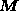from the 3-D projective space onto a point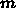of the 2-D projective plane. This projection can be written as a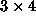homogeneous matrix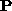of rank equal to 3 :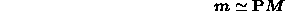where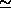is the equality up to a scale factor. If we restrict the 3-D projective space to the Euclidean space, then it is well known thatcan be written as :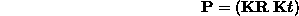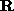andare the rotation and translation that link the camera frame to the 3-D Euclidean one. The most general form for the matrix of intrinsic parameters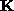is :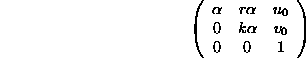whereis the horizontal scale factor, k is the ratio between the vertical and horizontal scale factors, r is the image skew and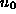and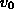are the image coordinates of the center of projection.

Bob Fisher
Mon Dec 7 13:48:06 GMT 1998